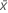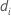# Methods and formulas for Randomization test for 2-sample means

Select the method or formula of your choice.

## Mean

A commonly used measure of the center of a batch of numbers. The mean is also called the average. It is the sum of all observations divided by the number of (nonmissing) observations.

### Notation

TermDescription
xiith observation
Nnumber of nonmissing observations

## Standard deviation (StDev)

The sample standard deviation provides a measure of the spread of your data. It is equal to the square root of the sample variance.

### Formula

If the column contains x 1, x 2,..., x N, with mean, then the standard deviation of the sample is:

### Notation

TermDescription
x i i th observationmean of the observations
N number of nonmissing observations

## Variance

The variance measures how spread out the data are about their mean. The variance is equal to the standard deviation squared.

### Notation

TermDescription
xiith observationmean of the observations
Nnumber of nonmissing observations

## Sum

### Notation

TermDescription
xi i th observation

## Minimum

The smallest value in your data set.

## Median

The sample median is in the middle of the data: at least half the observations are less than or equal to it, and at least half are greater than or equal to it.

Suppose you have a column that contains N values. To calculate the median, first order your data values from smallest to largest. If N is odd, the sample median is the value in the middle. If N is even, the sample median is the average of the two middle values.

For example, when N = 5 and you have data x1, x2, x3, x4, and x5, the median = x3.

When N = 6 and you have ordered data x1, x2, x3, x4, x5,and x6:

where x3 and x4 are the third and fourth observations.

## Maximum

The largest value in your data set.

## Average

### Notation

TermDescriptiondifference in means of the ith resamples
B number of resamples
N number of observations for one group in the original sample

## Standard deviation of the bootstrapping distribution

### Notation

TermDescriptionmean of the differences of the resamples
B number of resamplesdifference in means of the ith resample

## P-Value

### Formula

The calculation of the p-value depends on the alternative hypothesis.
• Mean less than hypothesized value:• Mean not equal to hypothesized value:• Mean greater than hypothesized value:### Notation

TermDescription
lnumber of bootstrap differences in means that are less than or equal to d
unumber of bootstrap differences in means that are greater than or equal to d
βnumber of resamples
nlnumber of bootstrap differences in means that are less than or equal to −d
nunumber of bootstrap differences in means that are greater than or equal to d
dsample difference in means
By using this site you agree to the use of cookies for analytics and personalized content.  Read our policy